# Mensuration Class 8 Notes- Chapter 11

According to the CBSE Syllabus 2023-24, this chapter has been renumbered as Chapter 9.

## What is Mensuration?

Mensuration is a branch of mathematics which mainly deals with the study of different kinds of Geometrical shapes along with their area, length, volume and perimeters. It is completely based on the application of both algebraic equations and geometric calculations. The results obtained by the Mensuration are considered very accurate. There are two types of geometric shapes:

To know more about Mensuration, visit here.

## Volume of Solids

### Volume of a 3D Object

Volume is the space occupied by the three dimensional object. It is a three-dimensional quantity.

### Volume of a Cuboid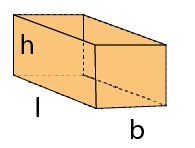Volume of a cuboid =l×b×h

where, l is the length, b is the breadth and h is the height of the cuboid.

### Volume of a Cube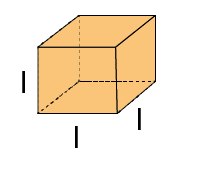Volume of a cube =l3

Where, l is the length of the each side of the cube.

### Volume of a Cylinder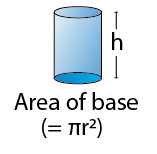Volume of the cylinder =πr2h

Where r is the radius of the base and h is the height of the cylinder.
To know more about Volume of Solids, visit here.

## Basics Revisited

### Introduction to Mensuration

Mensuration is the study of geometry that deals with the measurement of length, areas and volumes.

Perimeter is the total length or path of a given shape.
Area is the total region covered by the given shape.
Volume is the total space occupied by the given shape.

### Identifying Shapes and Areas of Different Regular Figures

Area of Square : side×side, perimeter : 4×side

Area of  Triangle : 1/2 (base×height), perimeter : a+b+c (sum of 3 sides)

Area of Parallelogram : base×height , perimeter : 2(length+breadth)

#### For more information on Area of Circle, watch the below video.## Trapezium

### Area of Trapezium by Division into Shapes of Known Area

Consider the trapezium where a and b are parallel sides, h is the height. Trapezium is divided into 3 parts : two triangles, one rectangle.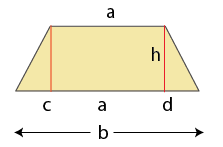Here h is the height, a and b are 2 parallel sides.

Area of trapezium = Area of 2 triangles + Area of rectangle
=[

$$\begin{array}{l}\frac{1}{2}\end{array}$$
×c×h+
$$\begin{array}{l}\frac{1}{2}\end{array}$$
×d×h]+[a×h]
=[
$$\begin{array}{l}\frac{1}{2}\end{array}$$
×c×h+
$$\begin{array}{l}\frac{1}{2}\end{array}$$
×d×h]+[
$$\begin{array}{l}\frac{1}{2}\end{array}$$

×2a×h]
[Multiplying and dividing by 2] =
$$\begin{array}{l}\frac{1}{2}\end{array}$$
×h(c+d+2a)

=
$$\begin{array}{l}\frac{1}{2}\end{array}$$
×h(c+d+a+a)

=
$$\begin{array}{l}\frac{1}{2}\end{array}$$
×h(b+a)
[ c + d + a = b]

Area of trapezium =

$$\begin{array}{l}\frac{1}{2}\end{array}$$
×h (sum of 2 parallel sides)

### Area of Trapezium by Finding the Area of a Triangle of Same Area

The area of the trapezium can be found out by dividing it into a triangle and a polygon.

Consider a trapezium WXYZ. Mark a midpoint A for side XY and join AZ. Cut the trapezium along AZ and obtain a ΔAZY
Flip the ΔAZY and place it as shown below. Now the new polygon is a triangle.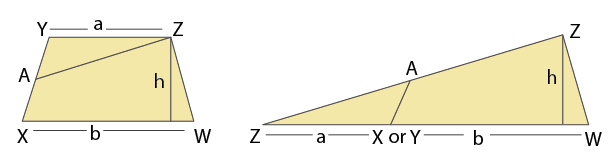We know that,
Area of a triangle=

$$\begin{array}{l}\frac{1}{2}\end{array}$$
×base×height
Substituting the values we get,
Area of a triangle=
$$\begin{array}{l}\frac{1}{2}\end{array}$$
×(a+b)×h

But the original polygon is a trapezium. So,

Area of a trapezium=

$$\begin{array}{l}\frac{1}{2}\end{array}$$
×(a+b)×h

#### For More Information On Area Of Trapezium, Watch The Below Video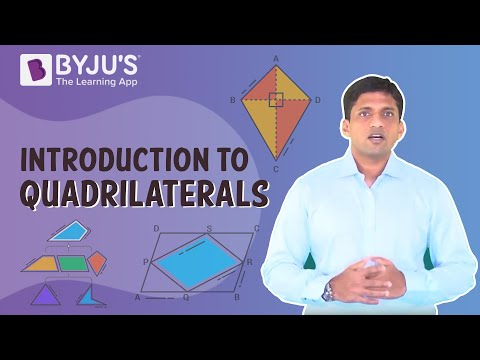### Area of a General Quadrilateral

Consider a quadrilateral ABCD. Draw diagonal AC. From B and D draw perpendiculars h1,h2 to AC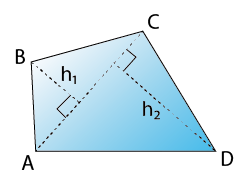Area of quadrilateral = Area of triangle ABC + Area of triangle ADC
=

$$\begin{array}{l}\frac{1}{2}\end{array}$$
×base×height+12×base×height
=(
$$\begin{array}{l}\frac{1}{2}\end{array}$$
×AC×h1)
+(
$$\begin{array}{l}\frac{1}{2}\end{array}$$
×AC×h2)
[Where, h1, h2 are the heights, AC is the base] =
$$\begin{array}{l}\frac{1}{2}\end{array}$$
×AC×(h1+h2)

=
$$\begin{array}{l}\frac{1}{2}\end{array}$$
×d×(h1+h2)
[ AC is a diagonal]

$$\begin{array}{l}\frac{1}{2}\end{array}$$
×d×(h1+h2)

where d is diagonal and h1,h2 are perpendicular drwan to a diagonal.

### Area of Rhombus

Area of rhombus =

$$\begin{array}{l}\frac{1}{2}\end{array}$$
×d1×d2,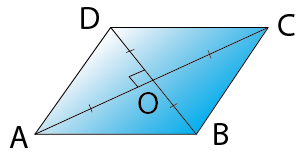where d1 and d2 are the diagonals.

### Area of Polygons

The area of any given polygon can be found by cutting the polygon into shapes whose area is known and adding the area of these shapes.

Some of the ways to find the area is shown below.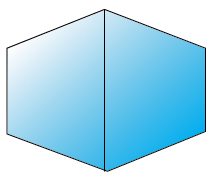Area of this polygon = area of 2 trapeziums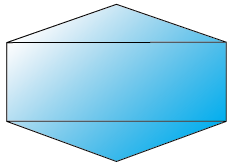Area of this polygon = Area of 2 triangles + Area of rectangle.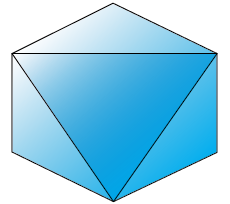Area of this polygon = Area of 4 triangles.

To know more about Areas of Different Regular Figures, visit here.

## Surface Area of Solids

### Solid Shapes

Solid shapes or solid figures are the three dimensional figures which have length, breadth and height. Using these, surface areas and volumes of these figures are found out.### Solids with a Pair or More of Identical Faces

Solids with a pair of identical faces are: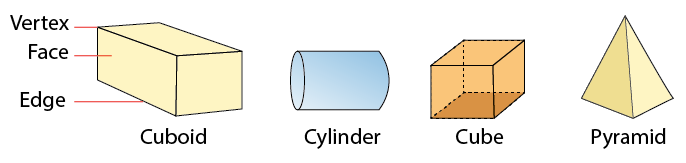### Surface Area of Solid Shapes

The surface area of the object is the total area occupied by the surface of the object.
or  surface area is simply the sum of the areas of the flat surfaces (called faces).

### Surface Area of a Cuboid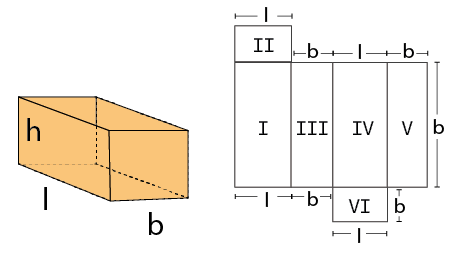Total Surface area of cuboid =2(lb+bh+lh)

Lateral Surface area of cuboid =2h(l+b)

Where, l is the length, b is the breadth and h is the height.

### Surface Area of a Cube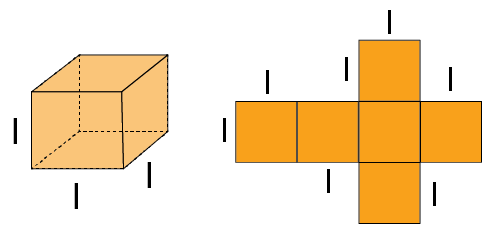Total Surface area of a cube =6l2

Lateral Surface area of a cube  =4l2

Where l is the length of each side of the cube.

### Surface Area of a Cylinder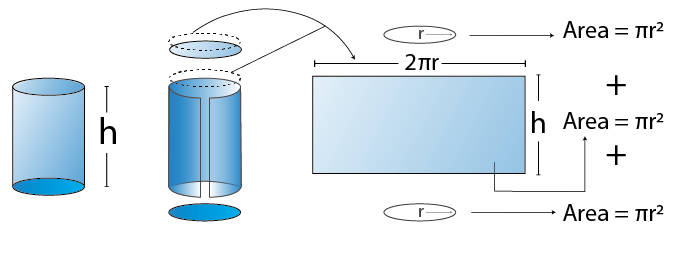Curved surface area of cylinder (C.S.A) =2πrh

Total Surface area of cylinder(T.S.A) =2πr(r+h)

Where, r is the radius of the cylinder and h is the height of the cylinder.

To know more about Surface Area and Volume, visit here.

## Summary of Mensuration

### Relation between Volume and Capacity

Volume is the total space occupied by an object. Volume is measured in cubic units,
Capacity refers to the maximum measure of an object’s ability to hold a substance, like a solid, a liquid or a gas. Capacity can be measured in almost every other unit, including liters, gallons, pounds, etc.
Eg : A bucket contains 9 litres of water, then its capacity is 9 litres.

## Frequently Asked Questions on CBSE Class 8 Maths Notes Chapter 11 Mensuration

Q1

### What is the meaning of a Trapezium?

A trapezium (also known as a trapeziod) is a flat 2D shape with four straight sides.

Q2

### What are the uses of mensuration in daily life?

1. Paint required to cover a certain area 2. Volume of soil needed to fill any ditch 3. Distance around a circular race track

Q3

### What is a Pyramid?

Pyramid is a large structure built especially in ancient Egypt that usually has a square base and four triangular sides meeting at a point and that contains tombs.